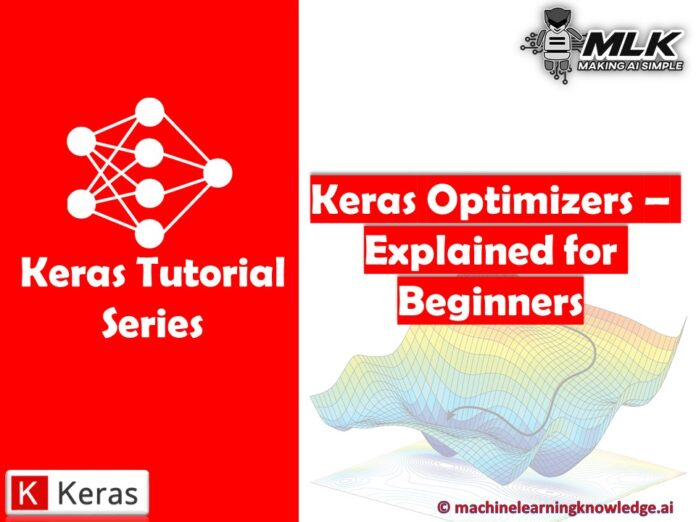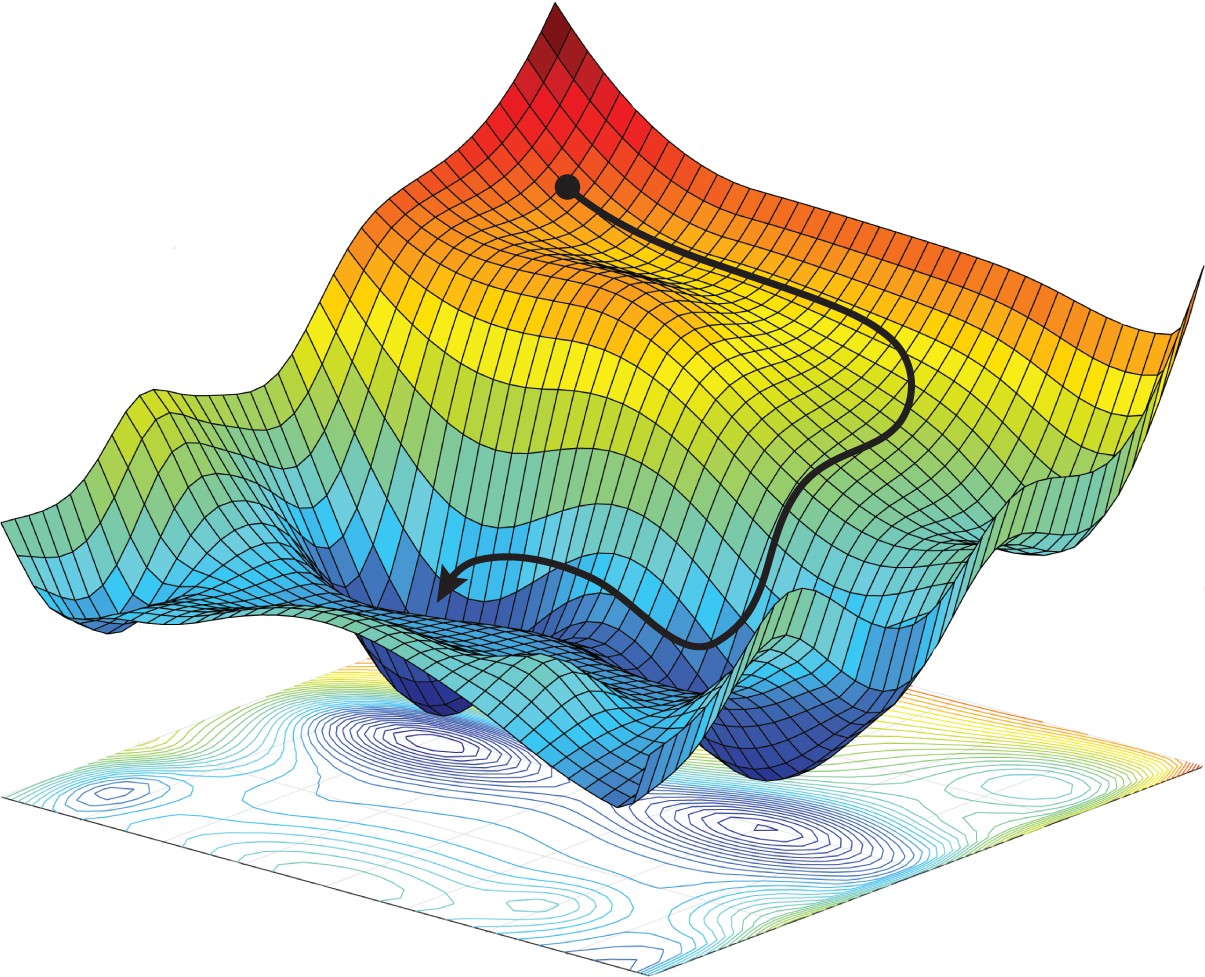# Keras Optimizers Explained with Examples for Beginners## Introduction

In this article, we will go through the tutorial for Keras Optimizers. We will explain why Keras optimizers are used and what are its different types. We will also cover syntax and examples of different types of optimizers in Keras for better understanding of beginners. At last, we’ll also compare the performance of the optimizers discussed in this Keras tutorial.

## Keras Optimizers Explained for Neural Network

Optimizers are not integral to Keras but a general concept used in Neural Network and Keras has out of the box implementations for Optimizers. But before going through the list of Keras optimizers we should first understand why optimizers.

When training a neural network, its weights are initially initialized randomly and then they are updated in each epoch in a manner such that they increase the overall accuracy of the network. In each epoch, the output of the training data is compared to actual data with the help of the loss function to calculate the error and then the weight is updated accordingly But how do we know how to update the weight such that it increases the accuracy?

This is essentially an optimization problem where the goal is to optimize the loss function and arrive at ideal weights. The method used for optimization is known as Optimizer. Gradient Descent is the most widely known but there are many other optimizers that are used for practical purposes and they all are available in Keras.Optimizers help Neural Network to convert on the lowest point on error surface known as minima (Source)

Keras provides APIs for various implementations of Optimizers. You will find the following types of optimizers in Keras –

1. SGD
2. RMSprop
8. Ftrl

### Keras Optimizer Examples of Usage

First of all, let us understand how we can use optimizers while designing neural networks in  Keras. There are two ways doing this –

1. Create an instance of the optimizer in Keras and use it while compiling the method.
2. Directly pass the string identifier for the Optimizer while compiling the method.

#### Example of 1st Method

In :
```from tensorflow import keras
from tensorflow.keras import layers

model = keras.Sequential()

model.compile(loss='categorical_crossentropy', optimizer=opt)
```

#### Example of 2nd Method

In :
```# pass optimizer by name: default parameters will be used
```

## Types of Keras Optimizers

Now we will understand different types of optimizers in Keras and their usage along with advantages and disadvantages.

### 1. Keras SGD Optimizer (Stochastic Gradient Descent)

SGD optimizer uses gradient descent along with momentum. In this type of optimizer, a subset of batches is used for gradient calculation.

#### Syntax of SGD in Keras

``````    tf.keras.optimizers.SGD
(learning_rate=0.01, momentum=0.0, nesterov=False, name="SGD", **kwargs)``````

#### Example of Keras SGD

Here SGD optimizer is imported from Keras library. We have also specified the learning rate in this example.

In :
```import numpy as np
import tensorflow as tf
```
In :
```opt = tf.keras.optimizers.SGD(learning_rate=0.1)
var = tf.Variable(1.0)
loss = lambda: (var ** 2)/2.0         # d(loss)/d(var1) = var1
step_count = opt.minimize(loss, [var]).numpy()
# Step is `- learning_rate * grad`
var.numpy()
```
Output:
`0.9`

### 2. Keras RMSProp Optimizer (Root Mean Square Propagation)

In the RMSProp optimizer, the aim is to ensure a constant movement of the average of square of gradients. And secondly, the division of gradient by average’s root is also performed.

#### Syntax of Keras RMSProp

``````tf.keras.optimizers.RMSprop(learning_rate=0.001, rho=0.9,
momentum=0.0, epsilon=1e-07, centered=False, name="RMSprop",**kwargs)``````

#### Example of Keras RMSProp (Root Mean Square Propagation)

The following example shows how keras library is used for implementing root mean square propagation optimizer.

In :
```opt = tf.keras.optimizers.RMSprop(learning_rate=0.1)
var1 = tf.Variable(10.0)
loss = lambda: (var1 ** 2) / 2.0    # d(loss) / d(var1) = var1
step_count = opt.minimize(loss, [var1]).numpy()
var1.numpy()
```
Output:
`9.683772`

The adam optimizer uses adam algorithm in which the stochastic gradient descent method is leveraged for performing the optimization process. It is efficient to use and consumes very little memory. It is appropriate in cases where huge amount of data and parameters are available for usage.

Keras Adam Optimizer is the most popular and widely used optimizer for neural network training.

``tf.keras.optimizers.Adam(learning_rate=0.001, beta_1=0.9 beta_2=0.999, epsilon=1e-07,amsgrad=False, name="Adam",**kwargs)``

#### Example of Keras Adam Optimizer

The following code snippet shows an example of adam optimizer.

In :
```opt = tf.keras.optimizers.Adam(learning_rate=0.1)
var1 = tf.Variable(10.0)
loss = lambda: (var1 ** 2)/2.0       # d(loss)/d(var1) == var1
step_count = opt.minimize(loss, [var1]).numpy()
# The first step is `-learning_rate*sign(grad)`
var1.numpy()
```
Output:
`9.9`

1. The continuous learning rate degradation during training.
2. It also solves the problem of the global learning rate.

``````tf.keras.optimizers.Adadelta(

Keras Adagrad optimizer has learning rates that use specific parameters. Based on the frequency of updates received by a parameter, the working takes place.

Even the learning rate is adjusted according to the individual features. This means there are different learning rates for some weights.

``````tf.keras.optimizers.Adagrad(learning_rate=0.001,